### Control Systems in Python - Part 1 - Bode and Step Response

I hate matlab with passion, yet sadly, nearly everyone uses it.  I'm a fan of Python and open source stuff so here's a simple article on how to do some common control systems stuff in Python.

### First we need to make sure the environment is setup.

1. Install IPython (or you can use any other python shell, but a unicode supported shell is preferred)
2. Install python-control (numpy, scipy)
3. Install sympy

These should do if your on Ubuntu/debian:

sudo apt-get install python-sympy python-numpy python-scipy python-matplotlib ipython

Then you need to install python control, see How to download and install python-control

## Intro to using Sympy

Open ipython and run the following:

import sympy
from sympy import *
sympy.init_printing()
s = Symbol('s')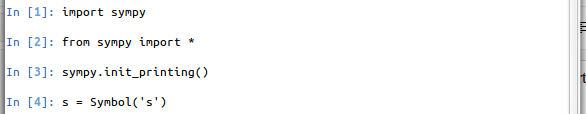Now we can do things like define transfer functions using the symbolic variable s.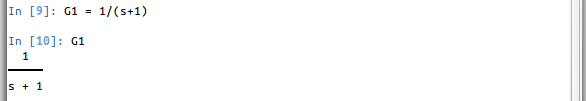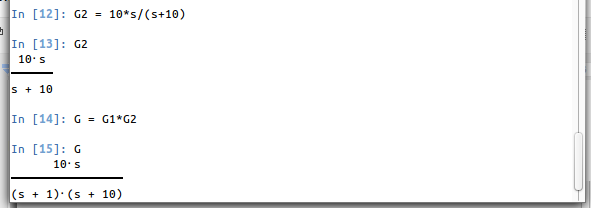We can expand the bottom using the .simplify() method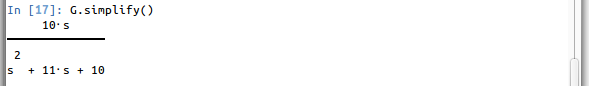and we can do something more complex like...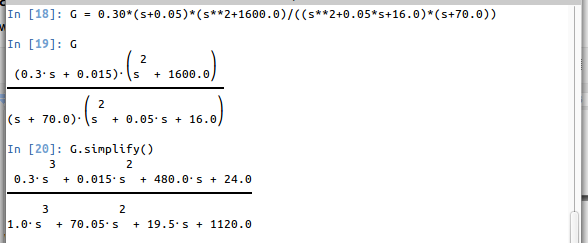which is really nice because it does all the multiplication for us... and it’s much prettier than matlab.

An example of a system in feedback form: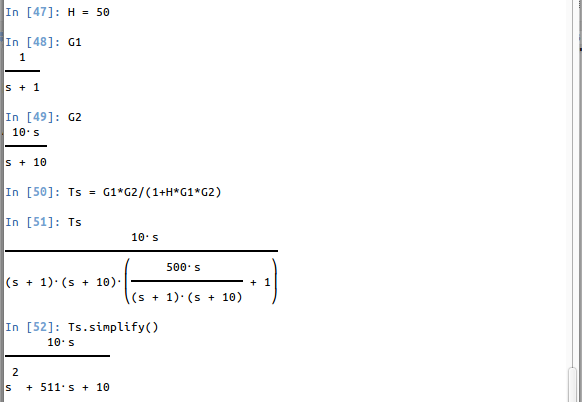Note: Use floats (number including a decimal )for all values you insert!

Doing Step Response Plots:
Okay cool, now let’s do something useful, add the following imports:

from control import matlab
import matplotlib.pyplot as plt

Copy or save and import this helper function (use %paste in iPython to enter it)

def stepResponse(Ts,*args,**kwargs):
num = Poly(Ts.as_numer_denom(),s).all_coeffs()
den = Poly(Ts.as_numer_denom(),s).all_coeffs()
tf = matlab.tf(map(float,num),map(float,den))
y,t = matlab.step([tf],*args,**kwargs)
plt.plot(t,y)
plt.title("Step Response")
plt.grid()
plt.xlabel("time (s)")
plt.ylabel("y(t)")
info ="OS: %f%s"%(round((y.max()/y[-1]-1)*100,2),'%')
try:
i10 = next(i for i in range(0,len(y)-1) if y[i]>=y[-1]*.10)
Tr = round(t[next(i for i in range(i10,len(y)-1) if y[i]>=y[-1]*.90)]-t[i10],2)
except StopIteration:
Tr = "unknown"
try:
Ts = round(t[next(len(y)-i for i in range(2,len(y)-1) if abs(y[-i]/y[-1])>1.02)]-t,2)
except StopIteration:
Ts = "unknown"

info += "\nTr: %s"%(Tr)
info +="\nTs: %s"%(Ts)
print info
plt.legend([info],loc=4)
plt.show()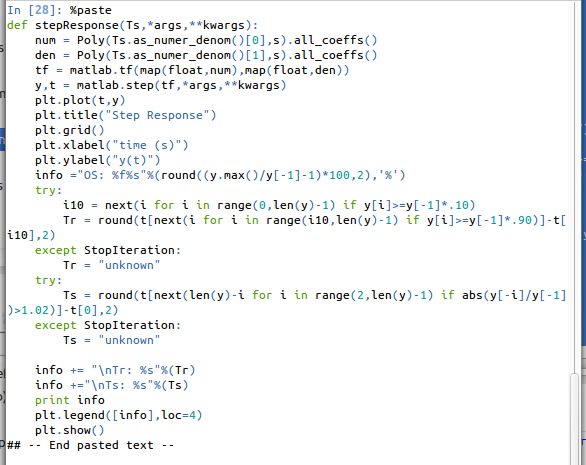Then run stepResponse(G), where Ts is the transfer function of the system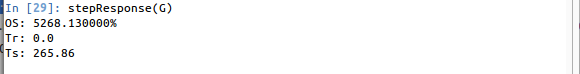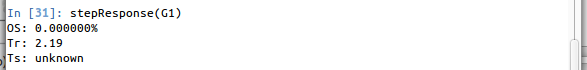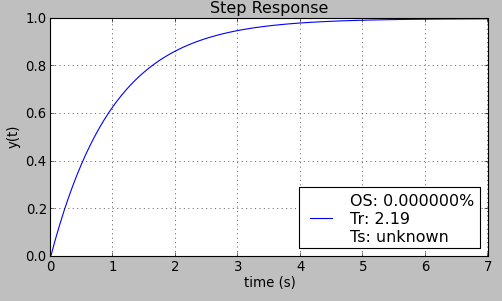# Doing Bode, Magnitude / Phase plots:

def freqResponse(Ts,*args,**kwargs):
num = Poly(Ts.as_numer_denom(),s).all_coeffs()
den = Poly(Ts.as_numer_denom(),s).all_coeffs()
tf = matlab.tf(map(float,num),map(float,den))
matlab.bode([tf],*args,**kwargs)
plt.show()

Then you just call freqResponse(ts) like: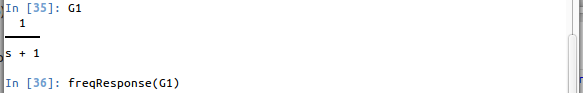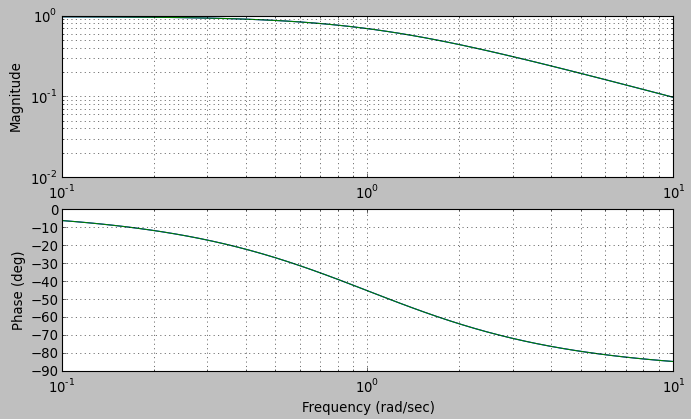You can also pass in arguments to the matlab.bode and matplotlib semilogx methods like: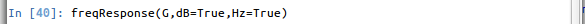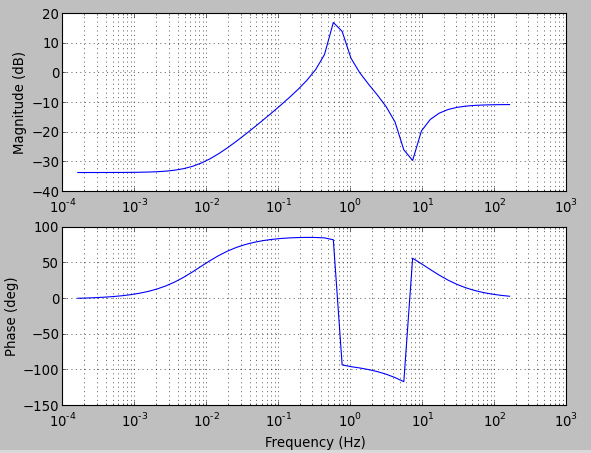which plots using dB and Hz scales.  You might notice this is pretty sketch looking, it actually missed a significant part of the magnitude plot, to smooth it out pass in your own omega values, like: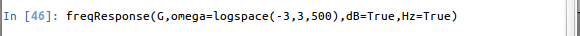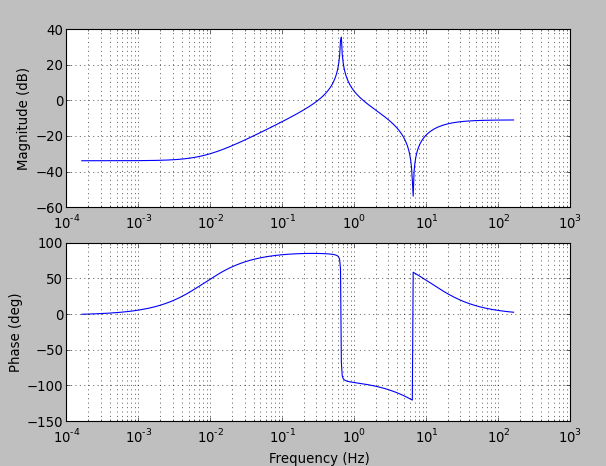which looks much better.

There’s lot more things you can do with the python-control library that isn’t implemented in the functions given here (such as plotting for various K values):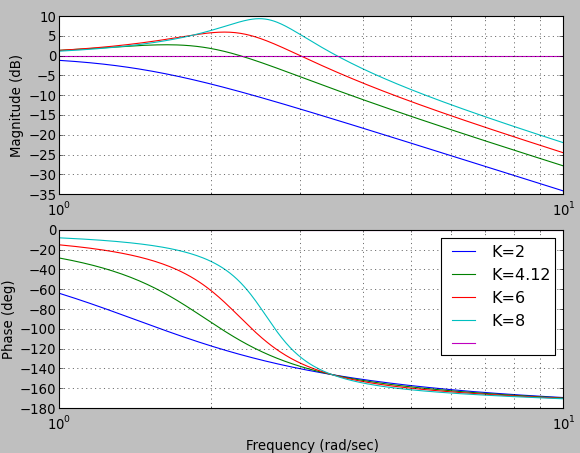Anyways that’s enough for now...

Good luck and have fun!

1.Hi Jairus,

Thanks for these posts with control systems + Python. I've been looking for an alternative to MATLAB to help confirm homework answers and this looks like it'll do well.

I had an issue initially with your stepResponse function - it seems the following line is invalid:

--
y,t = matlab.step([tf],*args,**kwargs)
--

I've removed the list for the tf argument and I'm now able to use the function. With the list matlab.step() doesn't return the expected yout/time lists with version 0.6.

Perhaps this is due to differing versions or something of that nature.

Bryon

2.This comment has been removed by the author.

3.4.yout, t = matlab.step(tf, *args, **kwargs) #should have no [ ] or?

5.Same I have tried with tf without [ ] and *args and **kwargs

6.Thanks for this post...and the others. !!!!!! Cheers

7.My code have it errors when i copy and paste. Would like to have a direct chat with you cos I'm trying to figure this out with my Lecturer and it will be great to finally get a solution